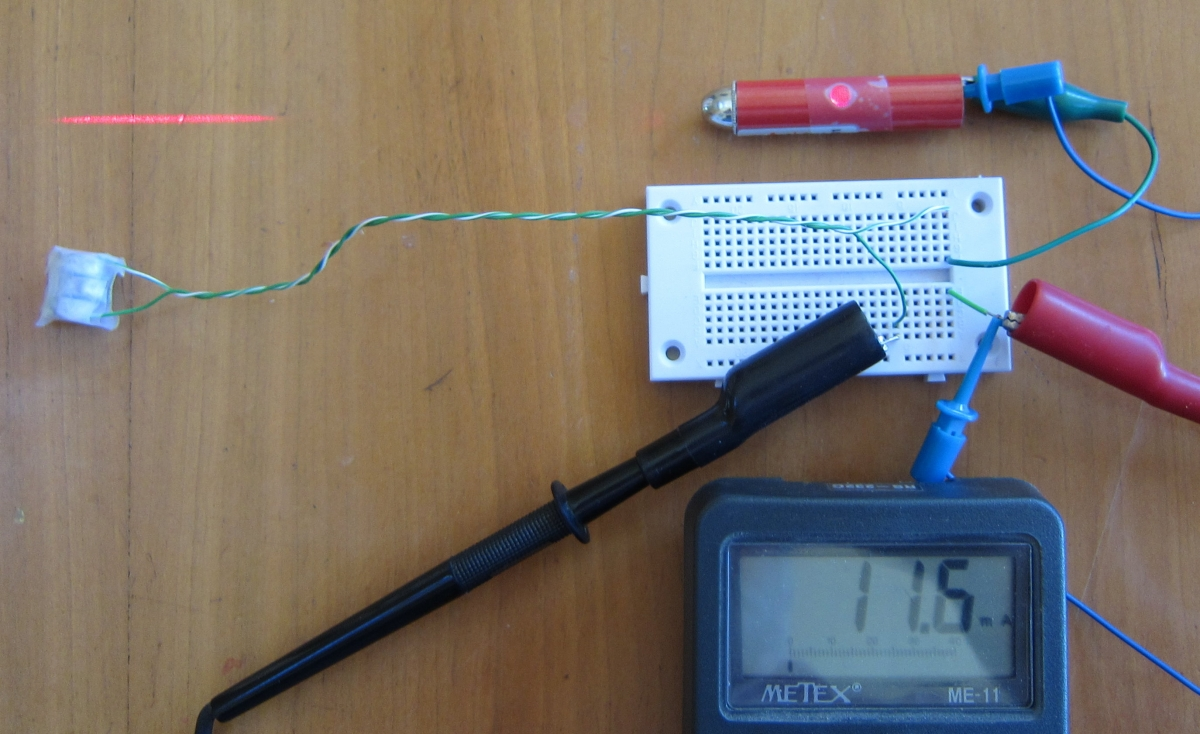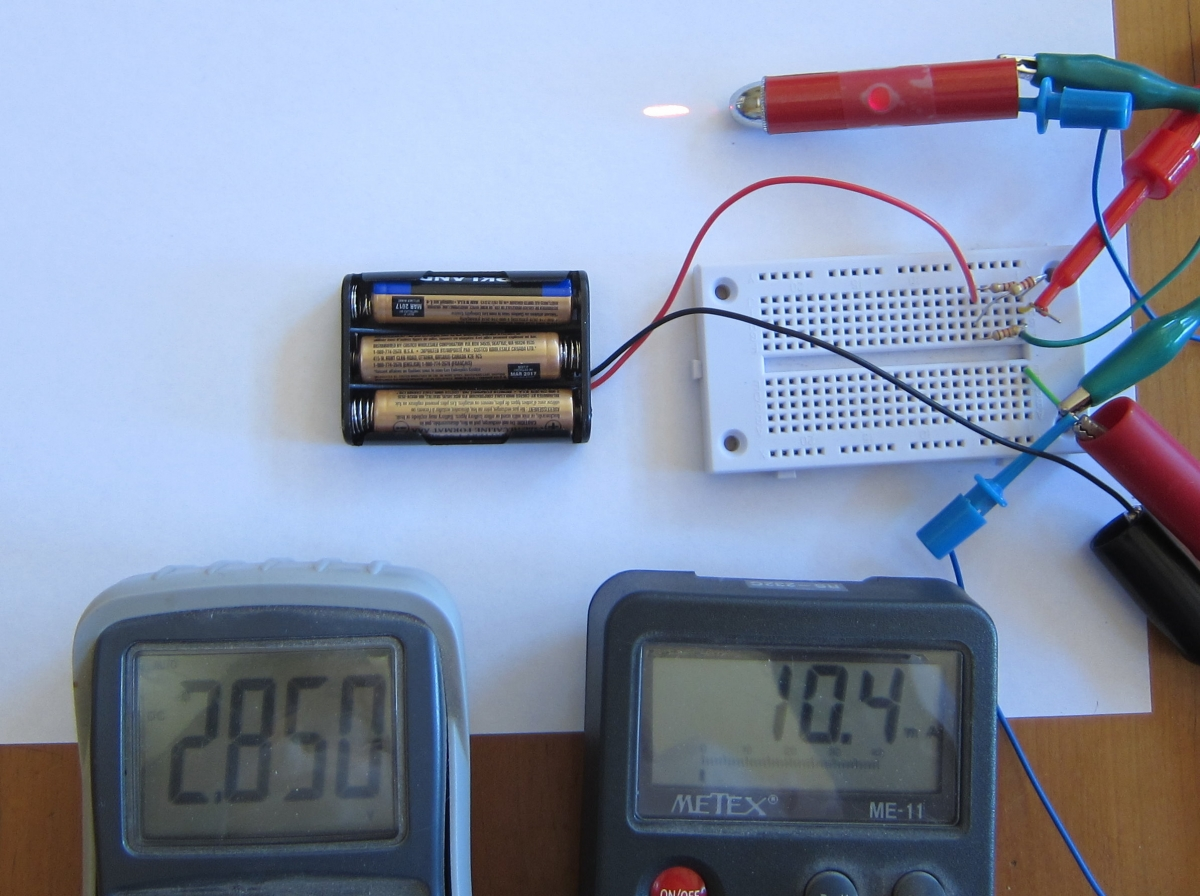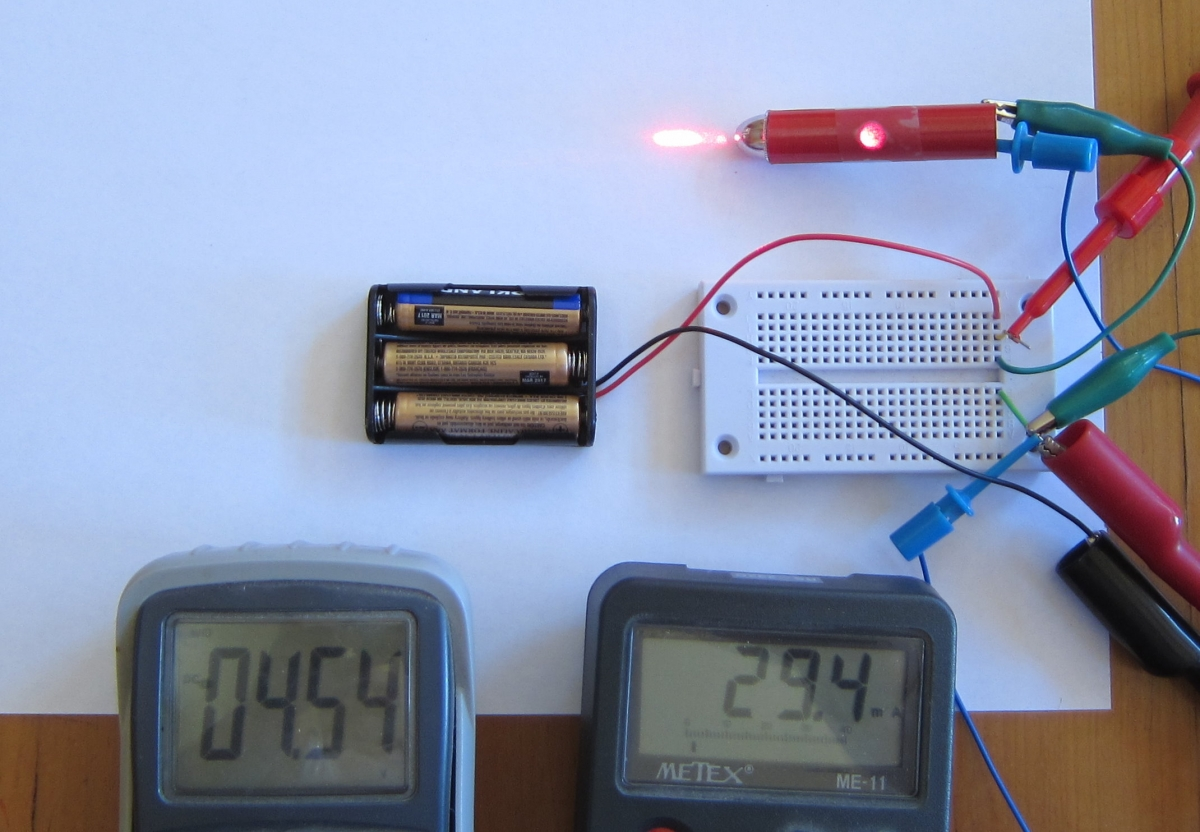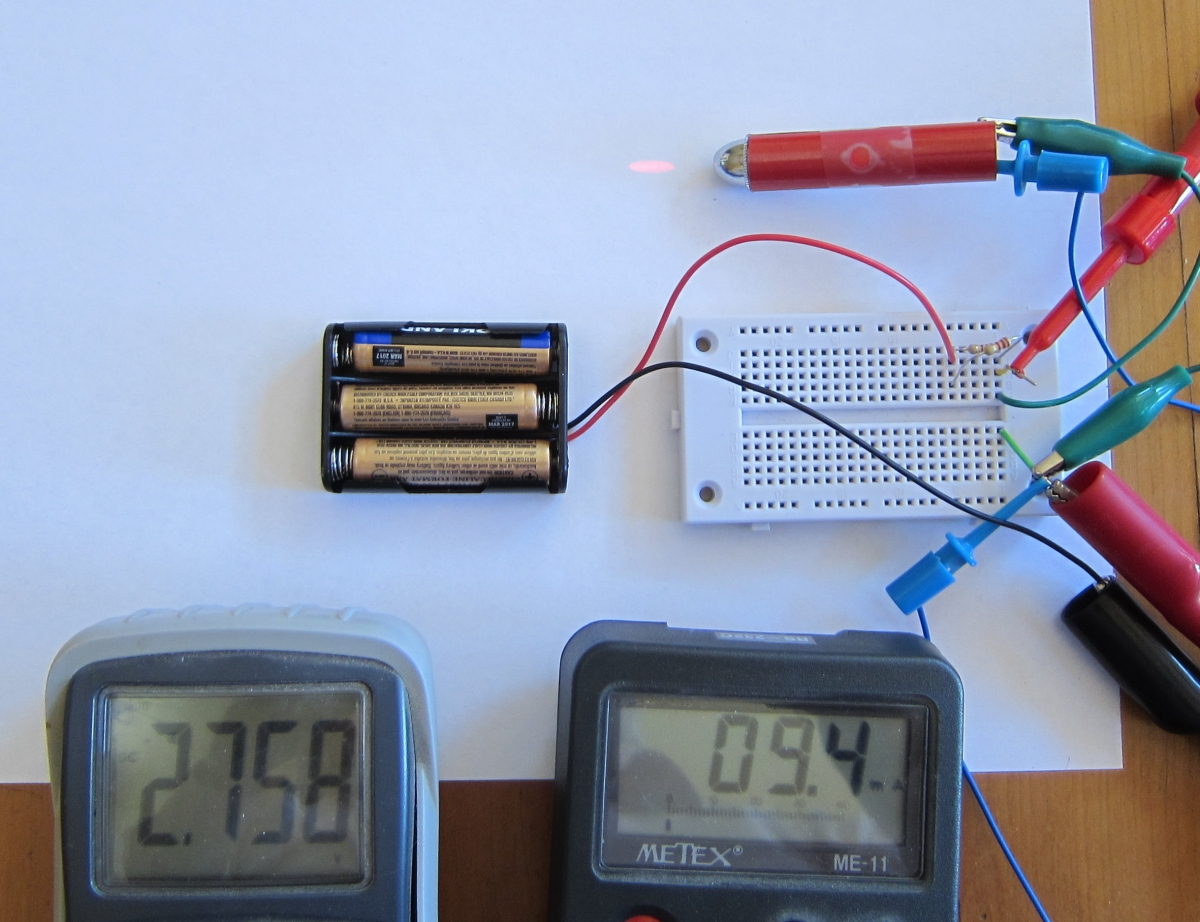# Controlling Electrical Current

When we were playing with LEDs, we tried to keep the current limited to 30 milliamperes. We got this number by looking at the specifications of the LED on the back of the package. But sometimes we get parts that have no detailed specifications, or we are re-using a part from some scavenged bit of electronics. How can we make sure we don't damage the part by using too much current?

We'll use a pocket laser pointer as an example. These little devices normally run on tiny button cell batteries. Little batteries like this might have the same output voltage (about 1.5 volts) as larger cells such as C or D flashlight batteries, but they can't supply the same amount of current as the larger cells. If we wanted to power our laser from a larger battery, in order to get longer battery life (or because the larger batteries are cheaper and easier to find), we might burn out the laser immediately when we connected it to the larger cells.

Pocket laser pointers are similar to LEDs in many ways. They use diode lasers, which are made of the same materials as LEDs. In fact, if we use low currents, the laser will just glow like an LED. But the laser diode chip is built in a special way, with the faces of the chip cut to make mirrors that bounce the light back and forth, allowing the light to be amplified until it is so bright that it escapes through the mirrors in a bright beam. This laser amplification only happens when the current is so high that the chip is very close to burning out. This makes controlling the current all the more important.

So let's see how much current the laser pointer uses when in normal operation. To do this, we need to put our meter in series with the batteries, as we did with the LEDs. We will simply use some tape to bind the three little batteries together, and some alligator clips to connect them to the little spring inside the laser's battery compartment, and the case of the laser. We use a bit of tape to hold down the power button so the laser stays on.The current (in this case, for this laser and these batteries) is a little under 12 milliamperes. Compared to our LEDs, it is using only about a third as much current.

If we have two meters, we can measure the current and the voltage drop across the laser diode at the same time.Here you can see I've made a few changes. Instead of the little batteries, I am using flashlight cells. I have added some resistance to keep the current to something close to what the laser normally encounters. I started with one resistor for safety, so the current would be too low to operate the laser. Then I added more resistors in parallel, to reduce the resistance (and thus increase the current) gradually. Adding resistors in parallel gives the electrons more ways to get through, so the current increases, and the resistance decreases.

The voltage drop is about 2.85 volts. When we learned Ohm's Law in the previous experiments, we saw that if we double the voltage going through a resistor, the current doubles also. Since this makes a straight line when we graph it, we say the relationship is linear. With a diode, the relationship is definitely not linear. As we double the voltage, the current through a diode goes up exponentially. This is what is really going on with that forward voltage drop. The diode seems to let all the current go through it once the voltage reaches the forward voltage drop level.

This is why it is important to limit the current using the resistors. Otherwise, the diode will allow all the current the battery can generate to go through it, and it will heat up until it fails.

Different diodes have different behavior with respect to voltage and current. They are exponential, but some more than others. This laser diode is not just a diode -- it has a resistor built into the circuit inside it, to limit the current from the tiny batteries. So as we increase the current, the forward voltage drop also increases.

Let's risk burning out our laser, and see if it can handle the same 30 milliamperes that our LED could.It can, although for how long we don't know. It may last years at its normal current, but only days or weeks at this higher current. But it is three times brighter than it was before.

Lasers have a voltage called the threshold voltage, below which there is not enough current for them to act as lasers. They act like ordinary LEDs below that current.Here I have lowered the current to 9.4 milliamperes, and suddenly the laser is much dimmer. When a laser beam hits a rough surface, you can see many tiny sparkles. These are called laser speckles, and are caused by the reflected light from the high points interfering with the reflected light from lower points on the rough surface. It is a hallmark of laser activity. But below the threshold current, we don't see the speckles. Our fancy laser is now just an ordinary LED. It is suddenly much dimmer because the light is not being amplified by the laser activity in the diode.

Once the voltage is above the threshold, the light output of the laser is linear with the current. So if we let three times the current go through, then the laser will be three times brighter. It looks like the manufacturers of this laser pointer kept the current very close to the threshold. This lowers the brightness (making the laser safer to use as a toy) and increases the battery life.Here is the laser operating at 60 milliamperes. It is well over 5 times as bright as when using the normal batteries. I had to use a nine-volt battery to get to this current level.

The nine-volt battery will only last an hour or so at a discharge rate of 60 milliamps before its voltage drops so much that it can't drive the laser. In the few minutes I was driving the laser at this rate, I could see the current steadily dropping as the battery discharged. And I would not expect the laser to last very long either.

We have been using resistors to limit the current in LEDs and lasers. I used resistors in parallel in this section in order to get a lower resistance. I could have simply found a resistor with a lower value, and used that. But when experimenting, it is handy to know how to quickly change resistances by putting resistors in series to increase the resistance, or in parallel to reduce it.Schematic symbol for a resistor

If we put one resistor after another, in series, then they both reduce the current flow. If they are both the same value, the resistance of both together is twice that of either one. In fact, you can place any number of different valued resistors in series, and the resistance is just the sum of all the resistances.

100 ohms

100 ohms = 200 ohms

Resistors allow fewer electrons to flow than a plain wire would. If we place two resistors together in parallel, the current has twice as many routes it can take. If the resistors have the same value, it is easy to see that together they will have half the resistance.

100 ohms = 50 ohms 100 ohms

That is as much as you probably need to know about resistors in parallel. But you might find it interesting to see how to calculate the resistance when you have several different values in parallel, so we have a separate section on that.

Before we leave off talking about resistors, there is one more neat trick we can do with them. If we have one voltage, say the voltage of a nine volt battery, but we need a smaller voltage, we can use two resistors to divide the voltage in half:

+9 volts 0 volts 4.5 volts 10,000 ohms 10,000 ohms

But it gets better. The voltage in the middle can be any voltage from zero to nine volts. We divide the resistance of the bottom resistor by the total resistance (both resistors added together). We multiply that by the input voltage to get the output voltage. So if we wanted three volts, we could make the bottom resistor  10,000 ohms, and the top resistor 20,000 ohms. 10,000 ohms divided by 30,000 ohms is 1/3rd, and 1/3rd of 9 volts is 3 volts. Or you can ask Google to do the arithmetic.

+9 volts 0 volts 3 volts 20,000 ohms 10,000 ohms

So why did I suddenly start using such large values for resistance? Look at what the circuit does. It connects one side of the battery to the other, through two resistors. If the resistance was low, a lot of current would flow. That would heat up the resistors, and they might even catch fire. But with 30,000 ohms of resistance, and 9 volts, Ohm's Law tells us that we will have a current of only 0.3 milliamperes. The resistors won't heat up much at all, and the battery won't quickly drain.

.